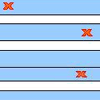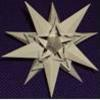# Resources tagged with: Iteration

Filter by: Content type:
Age range:
Challenge level:

### There are 22 results

Broad Topics > Patterns, Sequences and Structure > Iteration### Rain or Shine

##### Age 16 to 18Challenge Level

Predict future weather using the probability that tomorrow is wet given today is wet and the probability that tomorrow is wet given that today is dry.### Triangle Incircle Iteration

##### Age 14 to 16Challenge Level

Keep constructing triangles in the incircle of the previous triangle. What happens?### Infinite Continued Fractions

##### Age 16 to 18

In this article we are going to look at infinite continued fractions - continued fractions that do not terminate.### Difference Dynamics Discussion

##### Age 16 to 18

This article discusses what happens, and why, if you generate chains of sequences getting the next sequence from the differences between the adjacent terms in the sequence before it, eg (7, 2, 8, 3). . . .### First Forward Into Logo 10: Count up - Count Down

##### Age 11 to 18Challenge Level

What happens when a procedure calls itself?### Climbing Powers

##### Age 16 to 18Challenge Level

$2\wedge 3\wedge 4$ could be $(2^3)^4$ or $2^{(3^4)}$. Does it make any difference? For both definitions, which is bigger: $r\wedge r\wedge r\wedge r\dots$ where the powers of $r$ go on for ever, or. . . .### Stringing it Out

##### Age 14 to 16Challenge Level

Explore the transformations and comment on what you find.### Spirostars

##### Age 16 to 18Challenge Level

A spiropath is a sequence of connected line segments end to end taking different directions. The same spiropath is iterated. When does it cycle and when does it go on indefinitely?### Stretching Fractions

##### Age 14 to 16Challenge Level

Imagine a strip with a mark somewhere along it. Fold it in the middle so that the bottom reaches back to the top. Stetch it out to match the original length. Now where's the mark?### Archimedes Numerical Roots

##### Age 16 to 18Challenge Level

How did Archimedes calculate the lengths of the sides of the polygons which needed him to be able to calculate square roots?### Difference Dynamics

##### Age 14 to 18Challenge Level

Take three whole numbers. The differences between them give you three new numbers. Find the differences between the new numbers and keep repeating this. What happens?### Ford Circles

##### Age 16 to 18Challenge Level

Can you find the link between these beautiful circle patterns and Farey Sequences?### Converging Means

##### Age 14 to 16Challenge Level

Take any two positive numbers. Calculate the arithmetic and geometric means. Repeat the calculations to generate a sequence of arithmetic means and geometric means. Make a note of what happens to the. . . .### V-P Cycles

##### Age 16 to 18Challenge Level

Form a sequence of vectors by multiplying each vector (using vector products) by a constant vector to get the next one in the seuence(like a GP). What happens?### Peaches in General

##### Age 14 to 16Challenge Level

It's like 'Peaches Today, Peaches Tomorrow' but interestingly generalized.### The Golden Ratio, Fibonacci Numbers and Continued Fractions.

##### Age 14 to 16

An iterative method for finding the value of the Golden Ratio with explanations of how this involves the ratios of Fibonacci numbers and continued fractions.### Slippage

##### Age 14 to 16Challenge Level

A ladder 3m long rests against a wall with one end a short distance from its base. Between the wall and the base of a ladder is a garden storage box 1m tall and 1m high. What is the maximum distance. . . .### Route to Root

##### Age 16 to 18Challenge Level

A sequence of numbers x1, x2, x3, ... starts with x1 = 2, and, if you know any term xn, you can find the next term xn+1 using the formula: xn+1 = (xn + 3/xn)/2 . Calculate the first six terms of this. . . .### First Forward Into Logo 1: Square Five

##### Age 7 to 16Challenge Level

A Short introduction to using Logo. This is the first in a twelve part series.### Dalmatians

##### Age 14 to 18Challenge Level

Investigate the sequences obtained by starting with any positive 2 digit number (10a+b) and repeatedly using the rule 10a+b maps to 10b-a to get the next number in the sequence.### Loopy

##### Age 14 to 16Challenge Level

Investigate sequences given by $a_n = \frac{1+a_{n-1}}{a_{n-2}}$ for different choices of the first two terms. Make a conjecture about the behaviour of these sequences. Can you prove your conjecture?### Quorum-sensing

##### Age 16 to 18 ShortChallenge Level

This problem explores the biology behind Rudolph's glowing red nose.